# orthogonal polynomial

## polynomial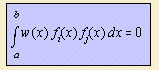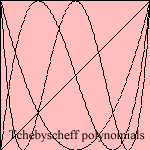According to algebraic theory systems of perpendicular (orthogonal) polynomials fi can be constructed, that span a so called function space. Orthogonality has the meaning of being zero of the inproducts <fi, fj> for i <> j. This function inproduct is defined as above mentioned integral, where w(x) is called the weight function.
Always true is that fi is a polynomial of degree i with the remarkable quality that all points of intersection with the x-axis are real and different, and are in the interval [a, b]. Some orthogonal polynomials have been given special attention, and were named to their explorer:

 - polynomial interval w(x) fn(x) abbreviation Hermite [-∞,∞]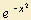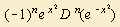Hn (x) Laguerre [0,∞]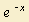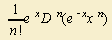Ln (x) Legendre [-1 , 1] 1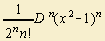Pn (x) Tchebyscheff 1) [-1 , 1]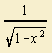?? Tn (x)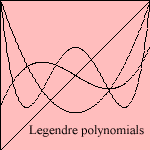The polynomials are also solutions of differential equations with the same name (equation of Hermite, Laguerre and so on), with whom I don't want you to torture.
The polynomials of Legendre are also called the
spherical functions of the first kind. A spherical function is a solution of the equation of Laplace 2), an equation to which a lot of theoretical problems in physics and astronomy can be reduced. The spherical term is derived from the solution method, where variables are split in spherical coordinates.
Working out this solution, derivatives of polynomials of Legendre, as function of cosφ, are formed.
These are called the associated polynomials of Legendre3).

From the Legendre polyonomial the Legendre trigonometric can be derived.

notes

1) Or: Tsjebysjev

2) Equation of Laplace: Δf = 0

3) See WolframMathWorld